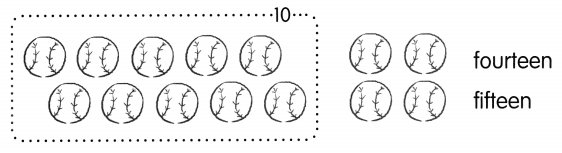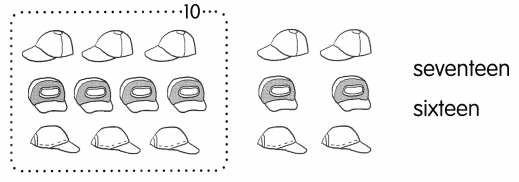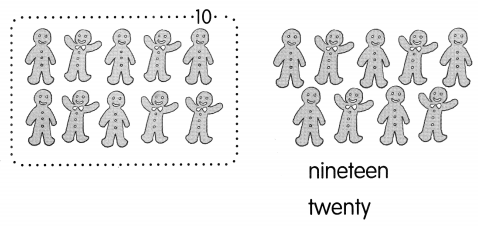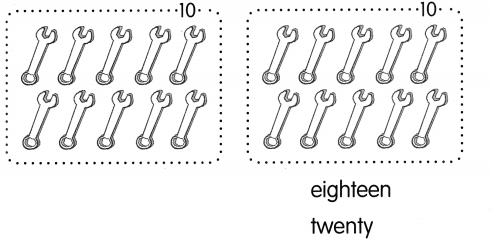# Math in Focus Grade 1 Chapter 7 Practice 1 Answer Key Counting to 20

This handy Math in Focus Grade 1 Workbook Answer Key Chapter 7 Practice 1 Counting to 20 detailed solutions for the textbook questions.

## Math in Focus Grade 1 Chapter 7 Practice 1 Answer Key Counting to 20

Write the numbers.

Example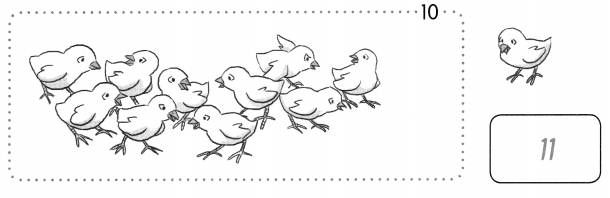Question 1.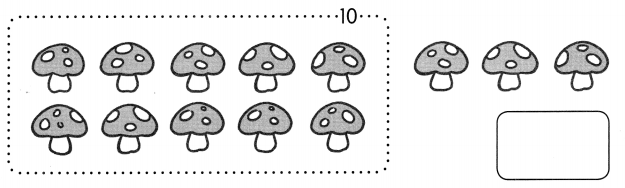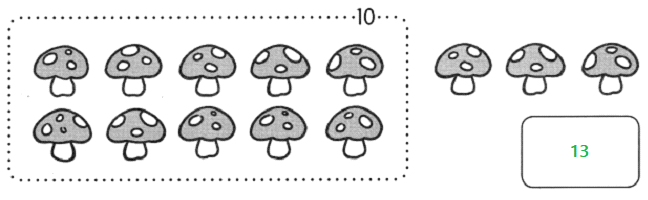Question 2.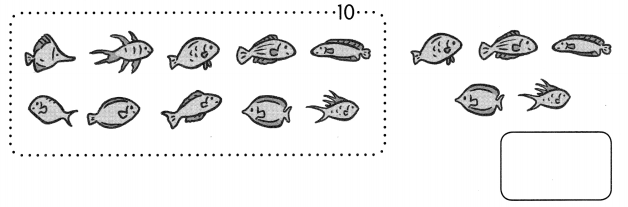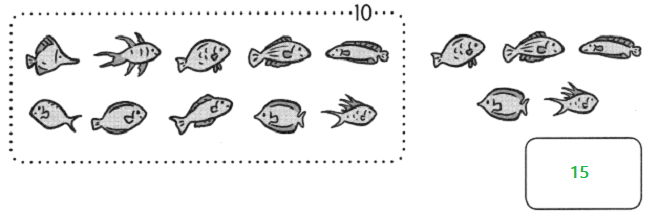Question 3.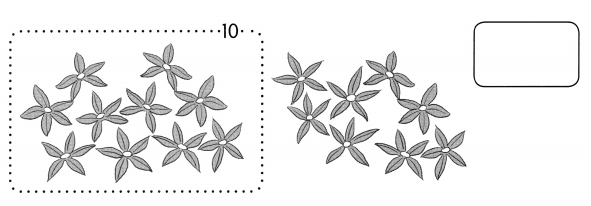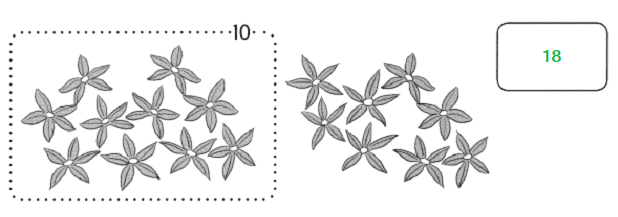Question 4.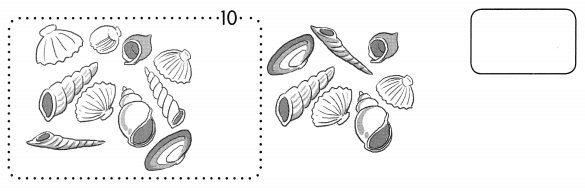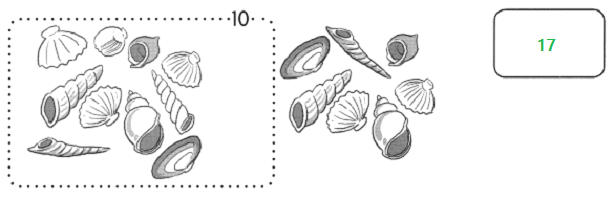Circle the ten. Color the rest. Write the numbers.

Example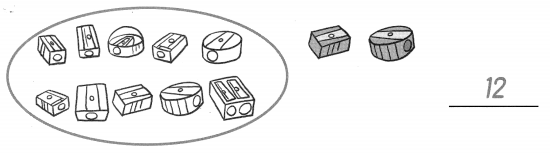Question 5.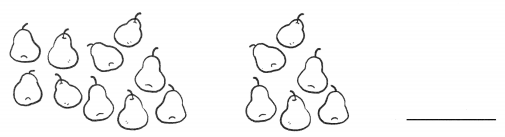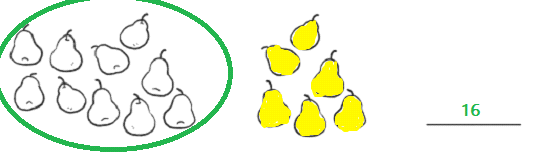Question 6.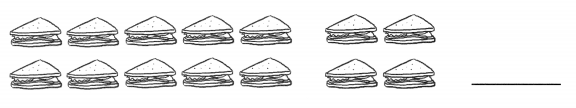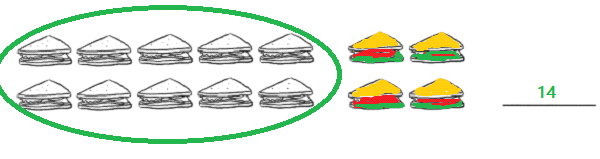Question 7.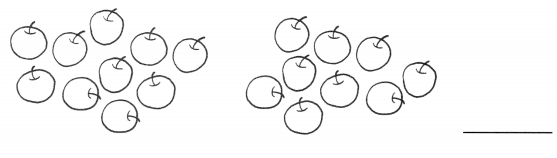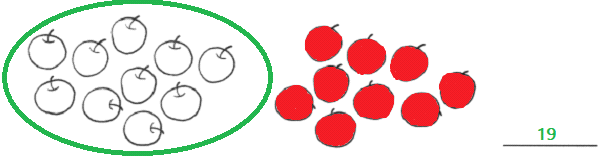Fill in the blanks.

Question 8.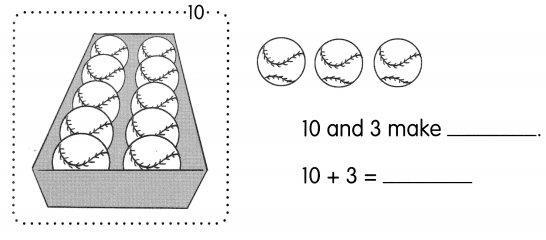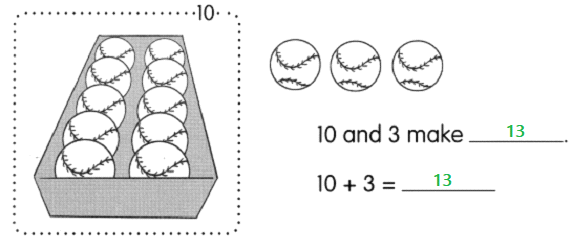Question 9.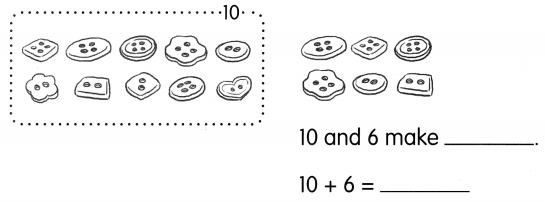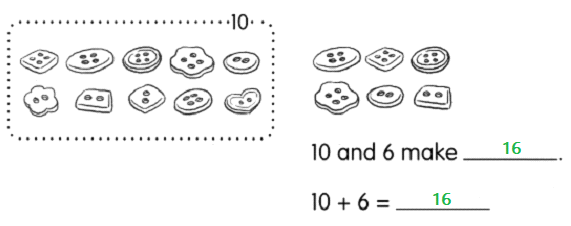Question 10.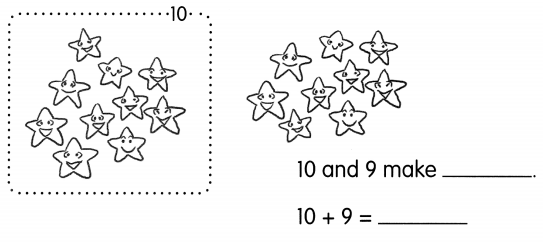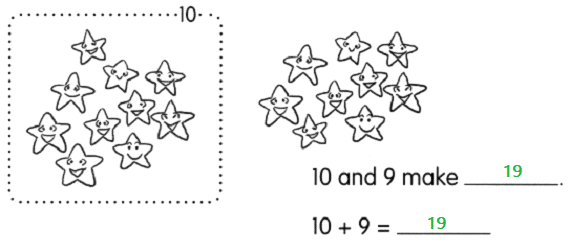Question 11.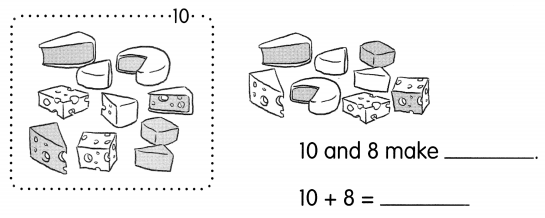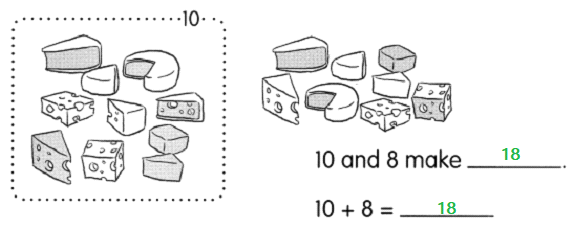Fill in the blanks with the correct number or word.

Question 12.
____ and ___ make 12.
Answer: 10 and 2 make 12.

Question 13.
___ and ___ make 15.
Answer: 10 and 5 make 15.

Question 14.
___ is ten and four.
Answer: 14 is ten and four.

Question 15.
____ is seven and ten.
Answer: 17 is seven and ten.

Fill in the blanks.

Question 16.
10 + 3 = ___

Question 17.
10 + 4 = ___

Question 18.
10 + 5 = ___

Question 19.
10 + 6 = ______

Question 20.
10 + 9 = ___

Question 21.
10 + 10 = ______

Question 22.
2 + 10 = ______

Question 23.
8 + 10 = ___

Count.

Circle the correct word.

Example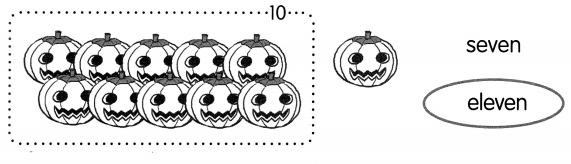Question 24.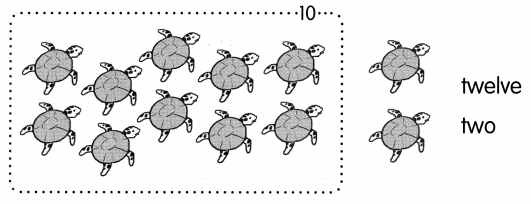Question 25.